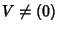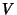## Jordan Decomposition Theorem

Letbe a finite dimensional Vector Space over the Complex Numbers, and letbe a linear operator on. Thencan be expressed as a Direct Sum of cyclic subspaces.

References

Gohberg, I. and Goldberg, S. ``A Simple Proof of the Jordan Decomposition Theorem for Matrices.'' Amer. Math. Monthly 103, 157-159, 1996.